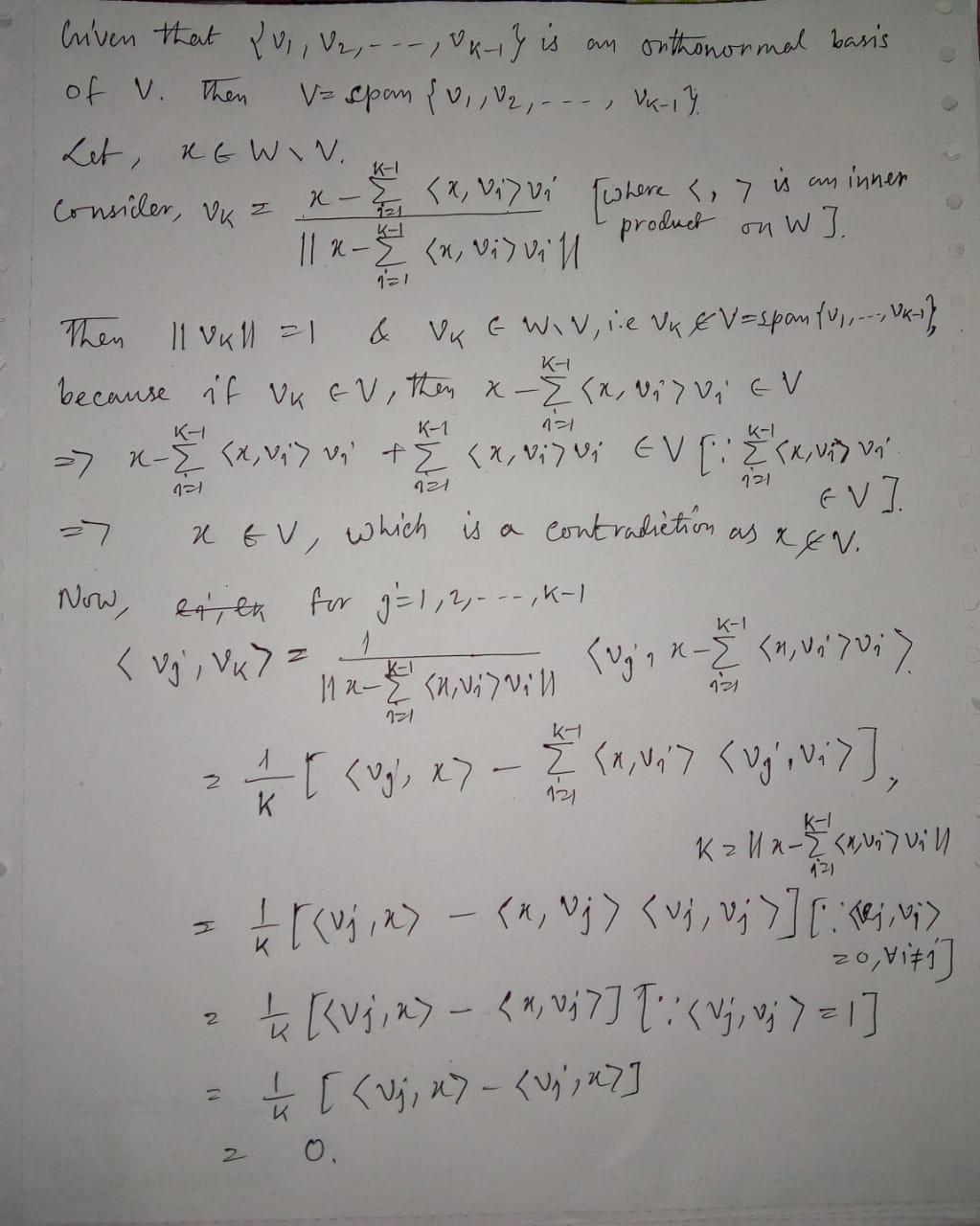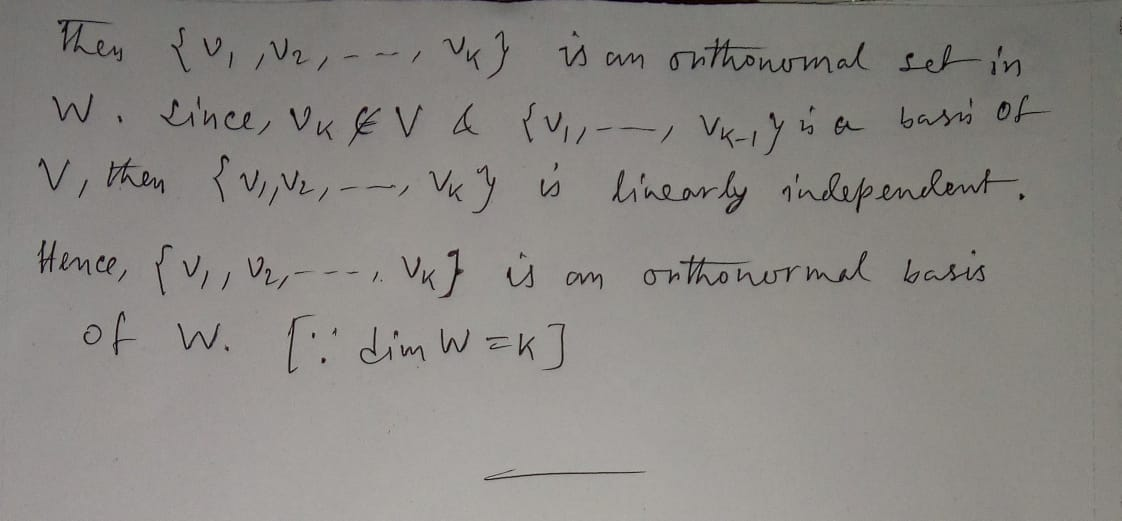# If V (dimension k-1) is a subspace of W (dimension K), and V has an orthonormal...

If V (dimension k-1) is a subspace of W (dimension K), and V has an orthonormal basis {v1,v2.....vk-1}. Work out a orthonormal basis of W in terms of that of V and the orthogonal complement of V in W.

Provide detailed reasoning.

## Solutions

##### Expert Solution## Related Solutions

##### Let V -Φ -> W be linear. Show that ker (Φ) is a subspace of V...
Let V -Φ -> W be linear. Show that ker (Φ) is a subspace of V and Φ (V) is a subspace of W.
##### Find a basis and the dimension of the subspace: V = {(x1, x2, x3, x4)| 2x1...
Find a basis and the dimension of the subspace: V = {(x1, x2, x3, x4)| 2x1 = x2 + x3, x2 − 2x4 = 0}
##### Determine whether or not W is a subspace of V. Justify your answer. W = {p(x)...
Determine whether or not W is a subspace of V. Justify your answer. W = {p(x) ∈ P(R)|p(1) = −p(−1)}, V = P(R)
##### Let V be a vector space of dimension 1 over a field k and choose a...
Let V be a vector space of dimension 1 over a field k and choose a fixed nonzero element voe V, which is therefore a basis. Let W be any vector space over k and let woe W be an arbitrary vector. Show that there is a unique linear transformation T: V → W such that T(v)= wo. [Hint: What must T(Avo) be?)
##### Let W be a subspace of Rn. Prove that W⊥ is also a subspace of Rn.
Let W be a subspace of Rn. Prove that W⊥ is also a subspace of Rn.
##### consider the subspace W=span[(4,-2,1)^T,(2,0,3)^T,(2,-4,-7)^T] Find A) basis of W B) Dimension of W C) is vector...
consider the subspace W=span[(4,-2,1)^T,(2,0,3)^T,(2,-4,-7)^T] Find A) basis of W B) Dimension of W C) is vector v=[0,-2,-5]^T contained in W? if yes espress as linear combantion
##### Prove the following: Let V and W be vector spaces of equal (finite) dimension, and let...
Prove the following: Let V and W be vector spaces of equal (finite) dimension, and let T: V → W be linear. Then the following are equivalent. (a) T is one-to-one. (b) T is onto. (c) Rank(T) = dim(V).
##### 1. For a map f : V ?? W between vector spaces V and W to...
1. For a map f : V ?? W between vector spaces V and W to be a linear map it must preserve the structure of V . What must one verify to verify whether or not a map is linear? 2. For a map f : V ?? W between vector spaces to be an isomorphism it must be a linear map and also have two further properties. What are those two properties? As well as giving the names...
##### Let W be a discrete random variable and Pr(W = k) = 1/6, k = 1,...
Let W be a discrete random variable and Pr(W = k) = 1/6, k = 1, 2 ,....., 6. Define X = { W,  if W <= 3; 1,  if W >= 4; } and Y = { 3,  if W <= 3; 7 -W,  if W >= 4; } (a) Find the joint probability mass function of (X, Y ) and compute Pr(X +Y = 4). (b) Find the correlation Cor(X, Y ). Are X and Y independent? Explain.
##### Determine whether or not W is a subspace of R3 where W consists of all vectors...
Determine whether or not W is a subspace of R3 where W consists of all vectors (a,b,c) in R3 such that 1. a =3b 2. a <= b <= c 3. ab=0 4. a+b+c=0 5. b=a2 6. a=2b=3c